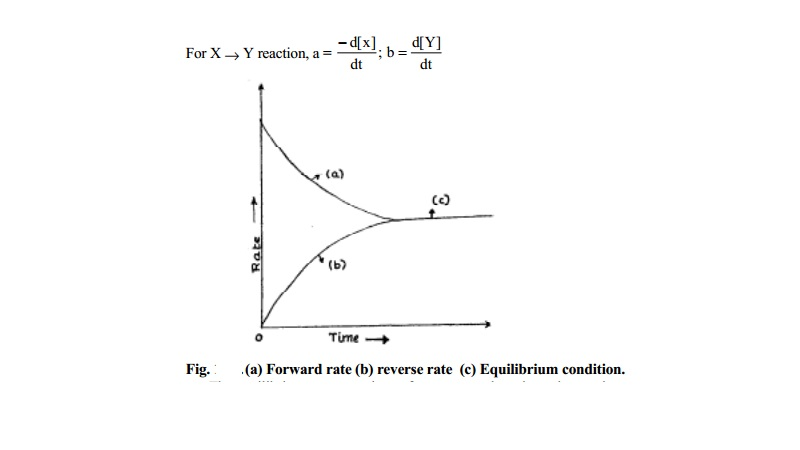Home | | Chemistry | | Chemistry | Scope and Nature of Chemical Equilibrium

# Scope and Nature of Chemical EquilibriumEquilibrium in Chemical Reactions : Consider a chemical reaction between A and B to form products C and D. After allowing sufficient period of time for the reaction, upon analyses, when A and B are absent in the reaction mixture, then the reaction is understood to be complete and only the presence of C and D will be detected.

Equilibrium in Chemical Reactions

Consider a chemical reaction between A and B to form products C and D. After allowing sufficient period of time for the reaction, upon analyses, when A and B are absent in the reaction mixture, then the reaction is understood to be complete and only the presence of C and D will be detected. For example, when sodium reacts with water, sodium hydroxide and hydrogen gas are produced, and the reverse reaction to form back the reactants never occurs even when the reaction vessel is a closed one. Reactions when go to completion and never proceed in the reverse direction are called as irreversible reactions. The chemical equations of such reactions are represented with a single arrow as A + B -- >  C + D.

For Example. 2 Na + 2 H2O -- >  2 NaOH + H2

However, even after allowing sufficient period of time for reaction, when the presence of A and B are always detected along with C and D, then such reactions are understood to be never complete.

For example, when H2 and I2 are reacted, 2 HI is formed. Initially the reaction proceeds to form HI until a certain period of time and with further increase in the reaction time, HI molecules dissociate to produce back H2 and I2 in such a way that, the reaction mixture always contain H2, I2 and HI for any length of time until external factors like temperature, pressure, catalyst etc. are applied. Reactions which never proceed to completion in both forward and backward direction are called as Equilibrium reactions.

The chemical equation of such reactions are represented as,

A + B -- > < -- C + D

Example H2 + I2 -- > < --- 2 HI

when both forward and reverse reaction rates are equal, the concentration of reactants and products do not change with any length of reaction time. Physical transformations of matter like change of solid to liquid states or liquid to vapour states also take place under equilibrium conditions with both the states of matter being present together. For example, at 0C, melting ice and freezing water are both present.

Scope of Chemical Equilibrium

Study of chemical equilibria possesses many scopes. The knowledge on whether the equilibrium lies in favour of reactants or products under certain experimental conditions is useful to increase yields in industrial processes, to establish the exact proton transfer equilibria in aqueous protein solutions. Since small changes in equilibrium concentration of hydrogen ion may result in protein denaturing and cell damage etc. This study is also useful or certain acids, bases and salts in water exist in ionic equilibria which control their use as buffers, colour indicators etc.

Reversible and Irreversible Reactions

A reaction which can go in the forward and backward direction simultaneously under the same conditions, is called a reversible reaction.

Nature of Chemical Equilibrium

The occurrence of chemical equilibrium is seen in reversible reactions only. Chemical equilibrium may be defined as the state of a reversible reaction when the two opposing reactions occur at the same rate and the concentration of reactants and products do not change with time. The true equilibrium of a reaction can be attained from both sides.

For X -- >  Y reaction,

a = - d[x] ;

b = d[Y] dt dtThe equilibrium concentrations of reactants and products do not change

with time. This is because, since the forward reaction rate equals with backward reaction rate as and when the products are formed, they react back to form the reactants in equal capacity. The equilibrium concentrations of reactants are different from their initial concentrations.

The equilibrium concentrations are represented by square brackets with subscript `eq' or as [ ]eq. Thus [A]eq denotes the equilibrium concentration of A in moles per litre. In modern practice, the subscript `eq' is not used.

Study Material, Lecturing Notes, Assignment, Reference, Wiki description explanation, brief detail

Related Topics